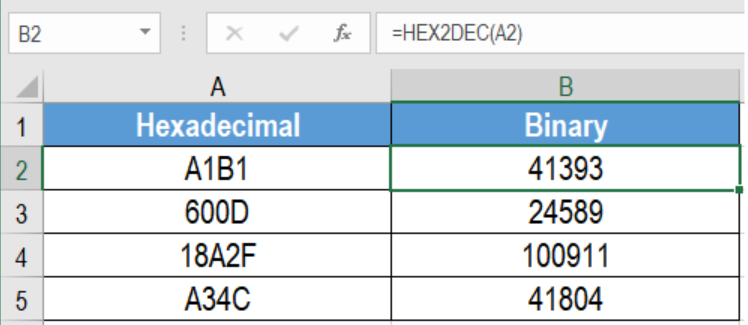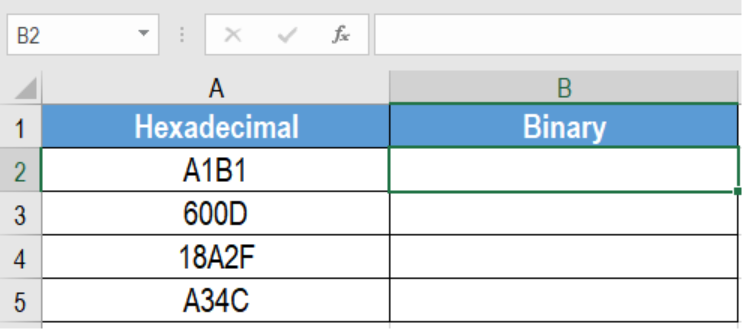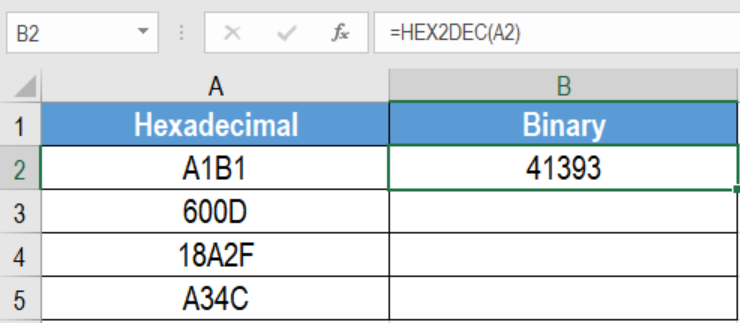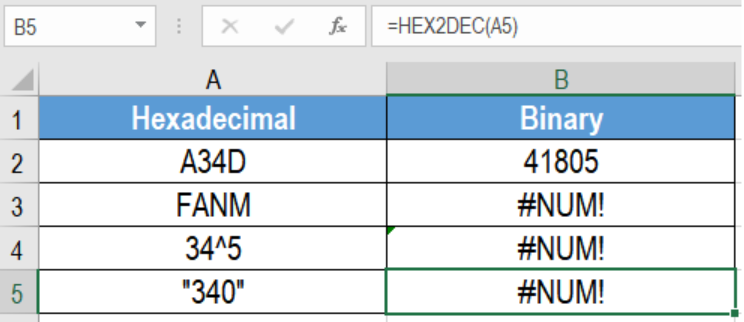Get instant live expert help with Excel or Google Sheets“My Excelchat expert helped me in less than 20 minutes, saving me what would have been 5 hours of work!”

#### Post your problem and you’ll get Expert help in seconds.

Your message must be at least 40 characters
Our professional Expert are available now. Your privacy is guaranteed.

# Excel HEX2DEC Function

Microsoft Excel offers some very effective functions to work with numbers. One such function is the HEX2DEC function. The HEX2DEC function converts a hexadecimal number to its decimal counterpart. In this tutorial, we will learn how to use the HEX2DEC function in Excel.Figure 1. Example of How to Use the HEX2DEC Function in Excel

## Syntax for HEX2DEC function

`=HEX2DEC(number)`

## Number

Required. This is the hexadecimal number that is converted to a decimal number.

## Setting up Data

The following example data set consisting of  hexadecimal numbers. The numbers are in column A. We will be converting these numbers to their decimal equivalents. The results will be in column B.Figure 2. Setting up Data to Use the HEX2DEC Function in Excel

In order to do that:

• We need to click cell B2 with the mouse.
• Assign the formula `=HEX2DEX(A2)` to the formula bar of cell B2.
• Press Enter.Figure 3. Applying the HEX2DEC Function in Excel

• Drag the fill handle from cell B2 from B2 to B5.

This will convert the hexadecimal numbers in column A to decimal numbers in column B.

## Notes

1. The number argument can have up to 10 characters (40 bits). The sign bit is the most significant bit of the number. The other 39 bits are used as magnitude bits. Excel represents negative numbers using two’s-complement notation.
2. The HEX2DEC function returns a #NUM! error if the number is not a hexadecimal number.
3. The function returns a #NUM! error if the number resembles >=3^23.Figure 4. Example of Common Errors with the HEX2DEC Function in Excel

Most of the time, the problem you will need to solve will be more complex than a simple application of a formula or function. If you want to save hours of research and frustration, try our live Excelchat service! Our Excel Experts are available 24/7 to answer any Excel question you may have. We guarantee a connection within 30 seconds and a customized solution within 20 minutes.